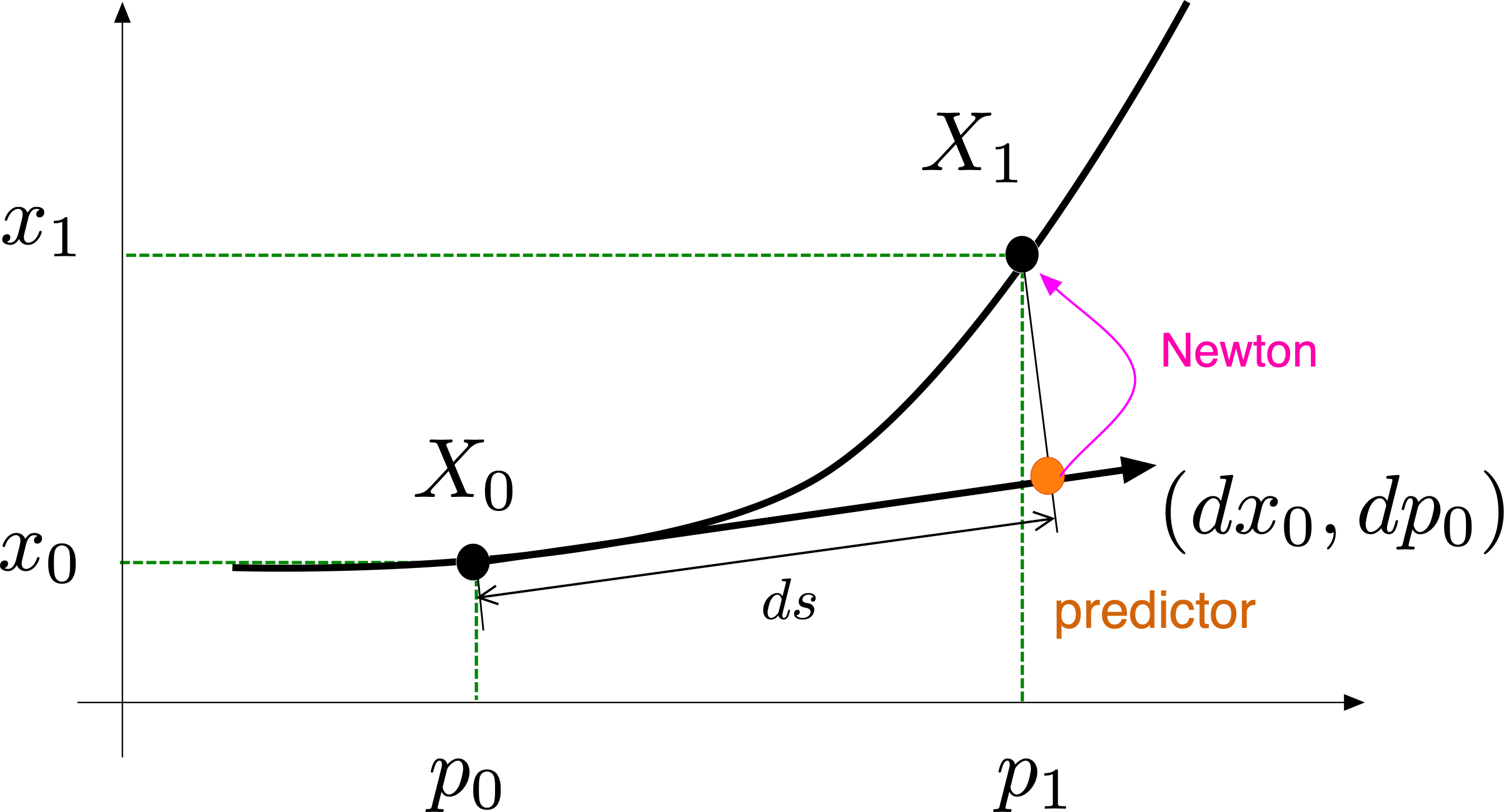# Pseudo arclength continuation

This is one of the various continuation methods implemented in BifurcationKit.jl. It is set by the option PALC(tangent = Bordered()) or PALC(tangent = Secant()) in continuation. See also PALC for more information.

For solving

$$$\mathbb R^n\ni F(x,p) = 0 \quad\tag{E}$$$

using a Newton algorithm, we miss an equation. The simplest way is to select an hyperplane in the space $\mathbb R^n\times \mathbb R$ passing through $(x_0,p_0)$:

$$$N(x, p) = \frac{\theta}{n} \langle x - x_0, dx_0\rangle + (1 - \theta)\cdot(p - p_0)\cdot dp_0 - ds = 0\tag{N}$$$

with $\theta\in[0,1]$ and where $ds$ is the pseudo arclength (see [Keller]).

Parameter θ

The parameter θ in the struct ContinuationPar is very important. It should be tuned for the continuation to work properly especially in the case of large problems where the $\langle x - x_0, dx_0\rangle$ component in the constraint might be favored too much. Also, large θs favour p as the corresponding term in the constraint $N$ involves the term $1-θ$.## Predictor

The possible predictors are listed in Predictors - Correctors.

## Corrector

The corrector is the newton algorithm for finding the roots $(x,p)$ of

$$$\begin{bmatrix} F(x,p) \\ N(x,p)\end{bmatrix} = 0\tag{PALC}$$$

## Linear Algebra

Let us discuss more about the norm and dot product. First, the option normC continuation specifies the norm used to evaluate the residual in the following way: $max(normC(F(x,p)), |N(x,p)|)<tol$. It is thus used as a stopping criterion for the corrector. The dot product (resp. norm) used in $N$ and in the (iterative) linear solvers is LinearAlgebra.dot (resp. LinearAlgebra.norm). It can be changed by importing these functions and redefining it. Note that by default, the $\mathcal L^2$ norm is used. These details are important because of the constraint $N$ which incorporates the factor length. For some custom composite type implementing a Vector space, the dot product could already incorporates the length factor in which case you should either redefine the dot product or change $\theta$.

The linear solver for the linear problem associated to (PALC) is set by the option linear_algo in continuation: it is one of Bordered linear solvers (BLS).

## Dot product

In the constraint $N$ above, the scalar product is in fact saved in BifurcationKit.jl as dotp(x,y) -> dot(x,y)/length(y). This is used in the bordered linear solvers associated to PALC. If you want to use your own dot product, you can pass

dotPALC = BK.DotTheta(mydot),

to continuation. Additionally, you may want to provide the linear operator P such that mydot(x,y) = dot(x, A*y), especially if you intend too use the linear solver MatrixBLS. We refer to BifurcationKit.DotTheta for more details.

## Step size control

Each time the corrector fails, the step size $ds$ is halved. This has the disadvantage of having lost Newton iterations (which costs time) and imposing small steps (which can be slow as well). To prevent this, the step size is controlled internally with the idea of having a constant number of Newton iterations per point. This is in part controlled by the aggressiveness factor a in ContinuationPar.

• Keller

Keller, Herbert B. Lectures on Numerical Methods in Bifurcation Problems. Springer, 1988